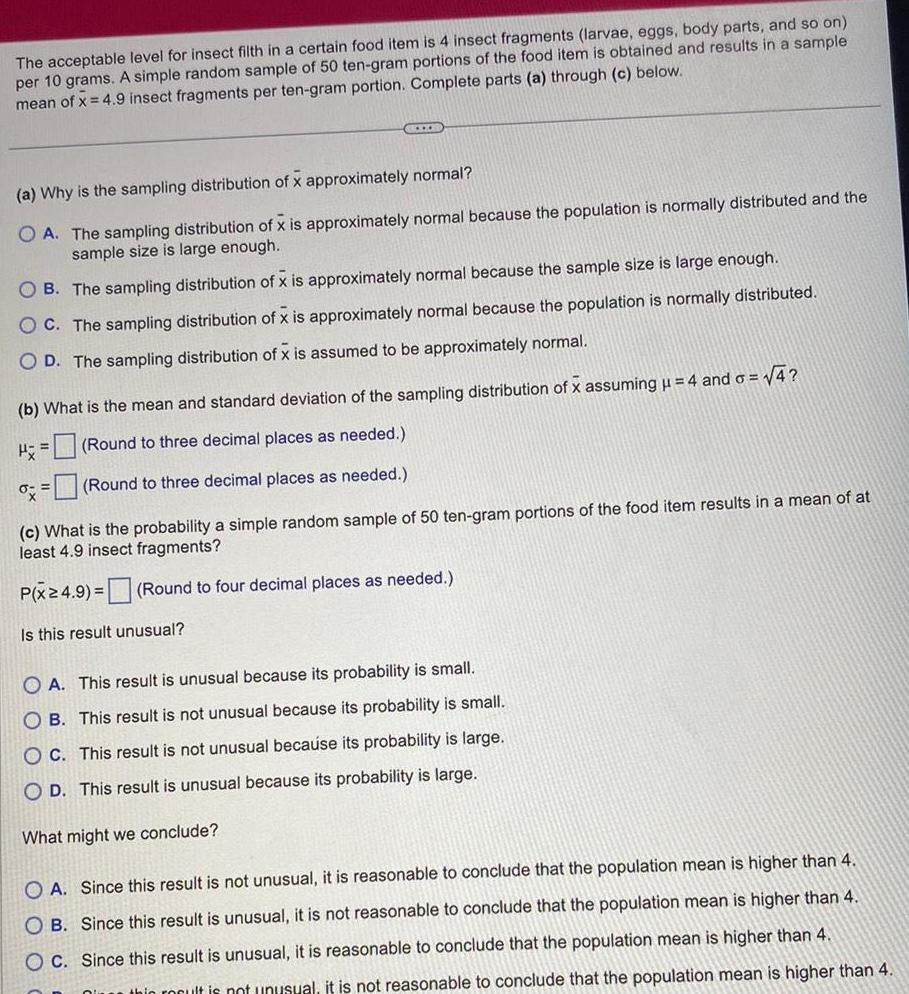Question:

# The acceptable level for insect filth in a certain food item

Last updated: 11/21/2023The acceptable level for insect filth in a certain food item is 4 insect fragments larvae eggs body parts and so on per 10 grams A simple random sample of 50 ten gram portions of the food item is obtained and results in a sample mean of x 4 9 insect fragments per ten gram portion Complete parts a through c below XXX a Why is the sampling distribution of x approximately normal O A The sampling distribution of x is approximately normal because the population is normally distributed and the sample size is large enough OB The sampling distribution of x is approximately normal because the sample size is large enough OC The sampling distribution of x is approximately normal because the population is normally distributed O D The sampling distribution of x is assumed to be approximately normal b What is the mean and standard deviation of the sampling distribution of x assuming 4 and o 4 H Round to three decimal places as needed Round to three decimal places as needed c What is the probability a simple random sample of 50 ten gram portions of the food item results in a mean of at least 4 9 insect fragments P x24 9 Round to four decimal places as needed Is this result unusual OA This result is unusual because its probability is small OB This result is not unusual because its probability is small OC This result is not unusual because its probability is large O D This result is unusual because its probability is large What might we conclude O A Since this result is not unusual it is reasonable to conclude that the population mean is higher than 4 OB Since this result is unusual it is not reasonable to conclude that the population mean is higher than 4 OC Since this result is unusual it is reasonable to conclude that the population mean is higher than 4 thic rocult is not unusual it is not reasonable to conclude that the population mean is higher than 4